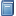Buchempfehlung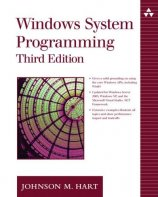Windows System Programming
Das Kompendium liefert viele interessante Informationen zur Windows-Programmierung auf Englisch. [Mehr Infos...]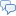FreeBASIC-Chat
Es sind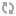Benutzer im FreeBASIC-Chat online.
(Stand:  )

# fb:porticula NoPaste

## antpap

 Uploader: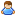ThePuppetMaster Datum/Zeit: 31.05.2008 15:54:41

```Dim Shared XShow as UByte = 0 Dim Shared XSW as UInteger = 600 Dim Shared XSH as UInteger = 150 Sub StatPrint(V_Text as String) Line (0, XSH * 4)-(XSW, XSH * 4 + 10), RGB(0, 0, 0), BF Locate (XSH * 4) \ 8 + 1, 1 Print V_Text Sleep 1, 1 End Sub screenres XSW, XSH * 4 + 20, 24 Dim TPic as UByte Ptr = ImageCreate(XSW, XSH, 24) Dim V as UInteger Dim C as UInteger Dim C2 as UInteger Dim X as UInteger Dim Y as UInteger Dim Z as UInteger Dim ZX as UInteger Dim ZY as UInteger Dim XX as UInteger Dim XY as UInteger Dim LX as UInteger Dim LY as UInteger Dim NP as UInteger Dim NX as UInteger Dim NY as UInteger Dim SX as UInteger Dim SY as UInteger Dim NF as UByte Dim CF as UInteger Dim XColorV() as UInteger Dim XColorC() as UInteger Dim XFontSize as UInteger = 98 Dim Font50 as UByte Ptr = ImageCreate(XFontSize * 26 * 2, XFontSize, 8) BLoad "font.bmp", Font50 If Font50 = 0 Then Screen 0: Print "Fehler beim laden des Bildes!": End -1 StatPrint("Buchstabentabelle fuer Erstnutzung vorbereiten...") For X = 0 to 51     StatPrint("Buchstabentabelle fuer Erstnutzung vorbereiten... [" & Str(X) & "]")     Line TPic, (0, 0)-(XSW, XSH), RGB(0, 0, 0), BF     Put TPic, (0, 0), font50, (X * XFontSize, 0)-((X + 1) * XFontSize, XFontSize), PSet     Put (0, 0), font50, (X * XFontSize, 0)-((X + 1) * XFontSize, XFontSize), PSet     C = 0     For ZX = 1 to XFontSize - 1         For ZY = 1 to XFontSize - 1             If (Point(ZX, ZY, TPic) and &HFFFFFF) <> 0 Then                 For NY = 1 to XFontSize                     For NX = ZX + 1 to XFontSize                         If (Point(NX, NY, TPic) and &HFFFFFF) <> 0 Then                             Put font50, (X * XFontSize, 0), TPic, (ZX, NY)-(XFontSize + ZX + 1, XFontSize + ZY + 1), PSet                             C = 1                             If XShow = 1 Then Sleep 500                         End If                         PSet (NX, NY), RGB(255, 0, 0)                         If C = 1 Then Exit For                     Next                     If C = 1 Then Exit For                 Next             End If             PSet (ZX, ZY), RGB(0, 255, 0)             If C = 1 Then Exit For         Next         If C = 1 Then Exit For     Next Next StatPrint("Lade Bild...") BLoad "../captcha/cap/10.bmp", TPic If TPic = 0 Then Print "Fehler!": end -1 Put (0, 0), TPic, (0, 0)-(XSW, XSH), pset StatPrint("Rauschfilter...") Dim XRauschBlockRaster as UInteger = 3 Dim XRauschschwelle as UInteger = 7 For X = 1 to XSW     For Y = 1 to XSH         V = Point (X, Y, TPic)         If V <> 0 Then             C = 0             For ZX = X to X + XRauschBlockRaster                 For ZY = Y to Y + XRauschBlockRaster                     If Point (ZX, ZY, TPic) = V Then C += 1                 Next             Next             If C > XRauschschwelle Then                 PSet (X, Y), V             Else: PSet (X, Y), RGB(0, 0 ,0)             End if         End If     Next     If XShow = 1 Then sleep 1, 1 Next Put TPic, (0, 0), Screenptr ,(0, 0) - (XSW, XSH), PSet StatPrint("Rasterscanner...") Dim XScanBlockRaster as UInteger = 3 Dim XScanSchwellwert as UInteger = 11 For X = 1 to XSW - XScanBlockRaster step XScanBlockRaster     For Y = 1 to XSH - XScanBlockRaster step XScanBlockRaster         Redim XColorV(XScanBlockRaster * XScanBlockRaster) as UInteger         Redim XColorC(XScanBlockRaster * XScanBlockRaster) as UInteger         C = 1         For ZX = X To X + XScanBlockRaster             For ZY = Y To Y + XScanBlockRaster                 V = Point (ZX, ZY)                 For Z = 1 to C                     If XColorV(Z) = V Then                         XColorC(Z) += 1                         V = 0                         Exit For                     End If                 Next                 If V > 0 Then                     C += 1                     XColorV(C) = V                     XColorC(C) = 1                     Exit For                 End If             Next         Next         ZX = 0         ZY = 0         For Z = 1 to C             If XColorV(Z) > 0 Then                 If XColorC(Z) > XScanSchwellwert Then                     If XColorC(Z) > ZX Then                         ZY = Z                         ZX = XColorC(Z)                     End If                 End If             End if         Next         If ZY > 0 Then             Line (X, XSH + Y)-(X + XScanBlockRaster, XSH + Y + XScanBlockRaster), XColorV(ZY), BF         Else: Line (X, XSH + Y)-(X + XScanBlockRaster, XSH + Y + XScanBlockRaster), 0, BF         End If     Next     If XShow = 1 Then sleep 1, 1 Next StatPrint("Objekterkennung / Mask-Rastnest...") Dim XSensTimeout as UInteger = 1000 Dim XSensSchwellwert as UInteger = 120 For X = XScanBlockRaster / 2 to XSW - XScanBlockRaster step XScanBlockRaster     For Y = XScanBlockRaster / 2 to XSH - XScanBlockRaster * 2 step XScanBlockRaster         V = Point (X, Y + XSH)         PSet (X, Y + XSH - 1), RGB(100, 100, 100)         If (V and &HFFFFFF) > 0 Then             C = 0             C2 = 0             For NX = X To 1 step -1                 If Point (NX, Y) <> V Then XX = NX: Exit For             Next             XY = Y             SX = XX             SY = XY             LX = XX + 1             LY = XY + 1             CF = 0             Line TPic, (0, 0)-(XSW, XSH), RGB(0, 0, 0), BF             Do                 NX = 1                 For Z = 1 to 8                     Select Case Z                         Case 1: If XX - 1   = LX and XY - 1 = LY Then NP = Z + 1: Exit For                         Case 2: If XX - 1   = LX and XY     = LY Then NP = Z + 1: Exit For                         Case 3: If XX - 1   = LX and XY + 1 = LY Then NP = Z + 1: Exit For                         Case 4: If XX       = LX and XY + 1 = LY Then NP = Z + 1: Exit For                         Case 5: If XX + 1   = LX and XY + 1 = LY Then NP = Z + 1: Exit For                         Case 6: If XX + 1   = LX and XY     = LY Then NP = Z + 1: Exit For                         Case 7: If XX + 1   = LX and XY - 1 = LY Then NP = Z + 1: Exit For                         Case 8: If XX       = LX and XY - 1 = LY Then NP = Z + 1: Exit For                     End Select                 Next                 NF = 0                 For Z = NP to 16                     Select Case Z                         Case 1, 9:  NX = XX - 1 :   NY = XY - 1                         Case 2, 10: NX = XX - 1 :   NY = XY                         Case 3, 11: NX = XX - 1 :   NY = XY + 1                         Case 4, 12: NX = XX     :   NY = XY + 1                         Case 5, 13: NX = XX + 1 :   NY = XY + 1                         Case 6, 14: NX = XX + 1 :   NY = XY                         Case 7, 15: NX = XX + 1 :   NY = XY - 1                         Case 8, 16: NX = XX     :   NY = XY - 1                     End Select                     If Point (NX, NY) = V Then                         PSet TPic, (NX, NY), V                         CF += 1                         NF = 1                         Exit For                     End If                 Next                 LX = XX                 LY = XY                 XX = NX                 XY = NY                 If XX = SY and XY = SY Then Exit Do                 C += 1                 If C > XSensTimeout Then Exit Do                 If CF = 0 Then                     C2 += 1                     If C2 > 10 Then Exit Do                 End If             Loop             CF = 0             C2 = 0             Put (0, XSH * 3), TPic, (0, 0)-(XSW, XSH), PSet             For ZX = 1 to XSW                 C2 = 0                 For ZY = 1 to XSH                     If Point (ZX, ZY, TPic) = V Then                         CF += 1                         For XY = ZY to XSH                             If Point (ZX, XY) <> V and Point (ZX, XY + 1) <> V Then                                 ZY = XY                                 Exit For                             Else                                 PSet TPic, (ZX, XY), V                                 PSet (ZX, XY + XSH * 3), V                                 CF += 1                                 If XShow = 1 Then sleep 1, 1                             End If                         Next                     End If                 Next             Next             If CF > XSensSchwellwert Then                 Put (0, XSH * 3), TPic, (0, 0)-(XSW, XSH), PSet                 Put (0, XSH * 2), TPic, (0, 0)-(XSW, XSH), Or             End If         End If         If XShow = 1 Then sleep 1, 1     Next     If XShow = 1 Then sleep 1, 1 Next StatPrint("Objekttrennung...") Dim XCutSchwellwert as UInteger = 5 C = 0 For X = 1 to XSW     C2 = 1     For Y = 1 to XSH         For Z = 1 to XCutSchwellwert             If (Point(X, Y + XSH * 2) and &HFFFFFF) > 0 Then C2 = 0: Exit For         Next         If C2 = 0 Then Exit For     Next     If C2 = 1 Then Line(X, XSH * 2)-(X, XSH * 3), RGB(255, 255, 255)     If XShow = 1 Then sleep 1, 1 Next Redim XColorC(XSW) as UInteger Redim XColorV(10) as UInteger CF = 0 StatPrint("Objektkorecktur / Rastertest...") For X = 1 to XSW     If (Point(X, XSH * 2) and &HFFFFFF) = 0 Then         PSet (X, XSH * 2 + 1), RGB(255, 0, 0)     Else: PSet (X, XSH * 2 + 1), RGB(0, 255, 0)     End If     If (Point(X, XSH * 2) and &HFFFFFF) = 0 Then         For XX = X to XSW             If (Point(XX, XSH * 2) and &HFFFFFF) <> 0 Then                 If XX - X > 20 Then                     C = 0                     For ZY = XSH * 2 to XSH * 3                         For ZX = X + 1 to XX - 1                             If (Point(ZX, ZY) and &HFFFFFF) <> 0 Then                                 Line(X, XSH * 2)-(XX, ZY), RGB(0, 255, 0), BF                                 Get (X + 1, ZY + 1)-(XX - 1, XSH * 3), TPic                                 C = 1                                 Exit For                             Else: PSet (ZX, ZY), RGB(255, 0, 0)                             End If                             If XShow = 1 Then Sleep 1, 1                         Next                         If C = 1 Then Exit For                     Next                     Line (X, XSH * 2 + 2)-(XX, XSH * 2 + 2), RGB(255, 255, 0)                     Line (0, XSH * 3)-(200, XSH * 4), RGB(0, 0, 0), BF                     Put (0, XSH * 3), TPic, (0, 0)-(XSW, XSH), PSet                     Redim XColorC(52) as UInteger                     For Z = 0 to 51                         StatPrint("Objektkorecktur / Rastertest... [" & Str(Z) & "]")                         XColorC(Z + 1) = 0                         Put (200, XSH * 3), font50, (Z * XFontSize, 0)-((Z + 1) * XFontSize, XFontSize), PSet                         Line (400, XSH * 3)-(600, XSH * 4), RGB(0, 0, 0), BF                         LX = 0                         For ZX = 1 to XFontSize - 1                             For ZY = 1 to XFontSize - 1                                 If (Point(ZX, XSH * 3 + ZY) and &HFFFFFF) <> 0 Then                                     LX += 1                                     If (Point(ZX + 200, XSH * 3 + ZY) and &HFFFFFF) <> 0 Then                                         PSet (ZX + 400, XSH * 3 + ZY), RGB(255, 0, 0)                                         XColorC(Z + 1) += 1                                     End If                                 End If                             Next                             If XShow = 1 Then sleep 1, 1                         Next                     Next                     C = 0                     C2 = 0                     For Z = 1 to 52                         If C < LX Then                             If XColorC(Z) > C Then                                 C = XColorC(Z)                                 C2 = Z                             End If                         End If                     Next                     CF += 1                     XColorV(CF) = C2                     Exit For                 Else: Line(X, XSH * 2)-(XX, XSH * 3), RGB(255, 255, 255), BF                 End If                 X = XX             End if         Next     End If     If XShow = 1 Then sleep 1, 1 Next Dim T as String For X = 1 to CF     Select Case XColorV(X)         Case 1 to 26: T += Chr(96 + XColorV(X))         Case 27 to 52: T += Chr(38 + XColorV(X))     End Select Next StatPrint("Fertig! Objekt-Vermutung: " & T) Do     sleep 1, 1 Loop until inkey() <> "" screen 0 end```